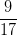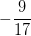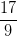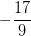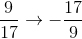# SSAT Upper Level Math : How to find the slope of perpendicular lines

## Example Questions

2 Next →

### Example Question #71 : Coordinate Geometry

Find the slope of a line that is perpendicular to the line with the equation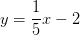.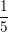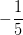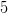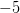Explanation:

Perpendicular lines have slopes that are negative reciprocals of each other. In other words, change the sign and flip the fraction around to find the slope of the line perpendicular to the given one.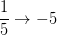### Example Question #72 : Coordinate Geometry

Find the slope of a line that is perpendicular to the line with the equation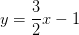.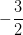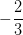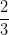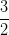Explanation:

Perpendicular lines have slopes that are negative reciprocals of each other. In other words, change the sign and flip the fraction around to find the slope of the line perpendicular to the given one.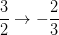### Example Question #73 : Coordinate Geometry

Find the slope of a line that is perpendicular to the line with the equation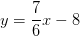.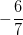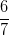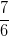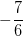Explanation:

Perpendicular lines have slopes that are negative reciprocals of each other. In other words, change the sign and flip the fraction around to find the slope of the line perpendicular to the given one.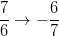### Example Question #74 : Coordinate Geometry

Find the slope of a line that is perpendicular to the line with the equation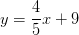.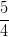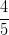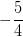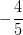Explanation:

Perpendicular lines have slopes that are negative reciprocals of each other. In other words, change the sign and flip the fraction around to find the slope of the line perpendicular to the given one.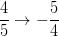### Example Question #75 : Coordinate Geometry

Find the slope of a line that is perpendicular to the line with the equation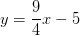.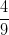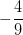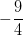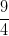Explanation:

Perpendicular lines have slopes that are negative reciprocals of each other. In other words, change the sign and flip the fraction around to find the slope of the line perpendicular to the given one.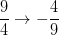### Example Question #76 : Coordinate Geometry

Find the slope of a line that is perpendicular to the line with the equation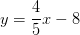.Explanation:

Perpendicular lines have slopes that are negative reciprocals of each other. In other words, change the sign and flip the fraction around to find the slope of the line perpendicular to the given one.### Example Question #77 : Coordinate Geometry

Find the slope of a line that is perpendicular to the line with the equation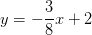.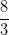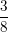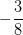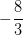Explanation:

Perpendicular lines have slopes that are negative reciprocals of each other. In other words, change the sign and flip the fraction around to find the slope of the line perpendicular to the given one.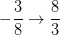### Example Question #81 : Lines

Find the slope of a line that is perpendicular to the line with the equation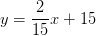.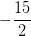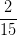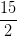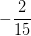Explanation:

Perpendicular lines have slopes that are negative reciprocals of each other. In other words, change the sign and flip the fraction around to find the slope of the line perpendicular to the given one.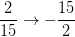### Example Question #82 : Lines

Find the slope of a line that is perpendicular to the line with the equation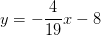.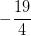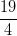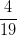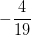Explanation:

Perpendicular lines have slopes that are negative reciprocals of each other. In other words, change the sign and flip the fraction around to find the slope of the line perpendicular to the given one.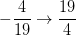### Example Question #83 : Lines

Find the slope of a line that is perpendicular to the line with the equation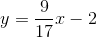.